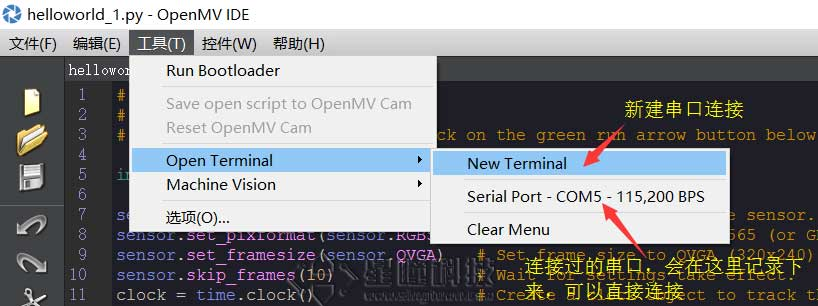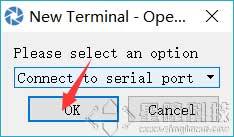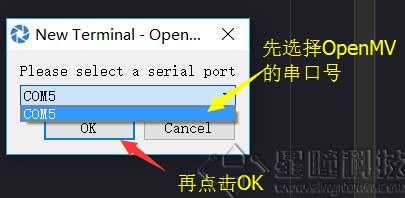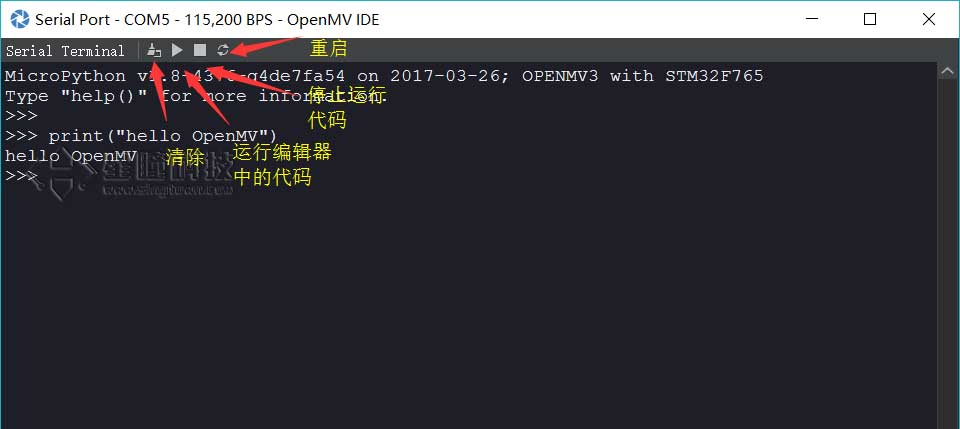# Python背景知识

## 使用python的好处

OpenMV可以使用Python来进行二次开发。有几个好处：

• 上手简单
• 不用考虑内存的申请和释放
• 有很多MicroPython库可以直接用（并不兼容PC上的Python模块）
• 开发团队开发了OpenMV的各种算法和模块可供调用

Python，是一个很常用的语言，在图像处理，机器学习，网络编程中都使用非常广。而且Python是一个非常容易上手的语言，如果你有其他语言编程基础（例如C，C++，Java），会更容易上手。

## REPL和串口

OpenMV的IDE自带一个串口助手，用于连接OpenMV，也可以连接其他的串口，比如Arduino，pyboard，esp8266。``````print("hello OpenMV!")
``````

``````hello OpenMV!
``````

## 输出

``````   print('hello, world')
``````
``````hello, world
``````

print()函数也可以接受多个字符串，用逗号“,”隔开，就可以连成一串输出：

``````  print('The quick brown fox', 'jumps over', 'the lazy dog')
``````
``````The quick brown fox jumps over the lazy dog
``````

print()会依次打印每个字符串，遇到逗号“,”会输出一个空格，因此，输出的字符串是这样拼起来的：

``````The quick brown fox jumps over the lazy dog
``````

print()也可以打印整数，或者计算结果：

``````  print(300)
``````
``````300
``````
``````  print(100 + 200)
``````
``````300
``````

``````   print('100 + 200 =', 100 + 200)
``````
``````100 + 200 = 300
``````

## 变量

``````  a = 123 # a是整数
print(a)
a = 'ABC' # a变为字符串
print(a)
``````

``````  int a = 123; // a是整数类型变量
a = "ABC"; // 错误：不能把字符串赋给整型变量
``````

## list列表

Python内置的一种数据类型是列表：list。list是一种有序的集合，可以随时添加和删除其中的元素。

`````` classmates = ['Michael', 'Bob', 'Tracy']
classmates
``````
``````['Michael', 'Bob', 'Tracy']
``````

``````  len(classmates)
``````
``````3
``````

`````` classmates
``````
``````'Michael'
``````
`````` classmates
``````
``````'Bob'
``````
`````` classmates
``````
``````'Tracy'
``````
`````` classmates
``````
``````Traceback (most recent call last):
File "<stdin>", line 1, in <module>
IndexError: list index out of range
``````

``````  classmates[-1]
``````
``````'Tracy'
``````

``````   classmates[-2]
``````
``````'Bob'
``````
``````   classmates[-3]
``````
``````'Michael'
``````
``````   classmates[-4]
``````
``````Traceback (most recent call last):
File "<stdin>", line 1, in <module>
IndexError: list index out of range
``````

list是一个可变的有序表，所以，可以往list中追加元素到末尾：

``````  classmates.append('Adam')
classmates
``````
``````  ['Michael', 'Bob', 'Tracy', 'Adam']
``````

``````  classmates.insert(1, 'Jack')
classmates
``````
``````  ['Michael', 'Jack', 'Bob', 'Tracy', 'Adam']
``````

``````  classmates.pop()
``````
``````  'Adam'
``````
``````  classmates
``````
``````  ['Michael', 'Jack', 'Bob', 'Tracy']
``````

``````   classmates = 'Sarah'
``````
`````` classmates
['Michael', 'Sarah', 'Tracy']
``````

list里面的元素的数据类型也可以不同，比如：

``````   L = ['Apple', 123, True]
``````

`````` L = []

len(L)

0
``````

## tuple元组

``````  classmates = ('Michael', 'Bob', 'Tracy')
``````

tuple的陷阱：当你定义一个tuple时，在定义的时候，tuple的元素就必须被确定下来，比如：

``````  t = (1, 2)

t

(1, 2)
``````

``````   t = (1)

t

1
``````

``````   t = (1,)

t

(1,)
``````

Python在显示只有1个元素的tuple时，也会加一个逗号,，以免你误解成数学计算意义上的括号。

## 条件判断

if语句的完整形式是：

``````if <条件判断1>:
<执行1>
elif <条件判断2>:
<执行2>
elif <条件判断3>:
<执行3>
else:
<执行4>
``````

``````age = 20
if age >= 6:
print('teenager')
elif age >= 18:
else:
print('kid')
``````

# 循环

Python的循环有两种，一种是for...in循环，依次把list或tuple中的每个元素迭代出来，看例子：

``````names = ['Michael', 'Bob', 'Tracy']
for name in names:
print(name)
``````

``````  Michael
Bob
Tracy
``````

``````  list(range(5))

[0, 1, 2, 3, 4]
``````

range(101)就可以生成0-100的整数序列，计算如下：

``````sum = 0
for x in range(101):
sum = sum + x
print(sum)
``````

``````sum = 0
n = 99
while n > 0:
sum = sum + n
n = n - 2
print(sum)
``````

===== 数据类型转换 =====

Python内置的常用函数还包括数据类型转换函数，比如int()函数可以把其他数据类型转换为整数：

``````>>> int('123')
123
>>> int(12.34)
12
>>> float('12.34')
12.34
>>> str(1.23)
'1.23'
>>> str(100)
'100'
>>> bool(1)
True
>>> bool('')
False
``````

===== 函数 =====

``````def power(x):
return x * x
``````

power(5)
25
power(15)
225

``````def power(x, n):
s = 1
while n > 0:
n = n - 1
s = s * x
return s
``````

power(5, 2)
25
power(5, 3)
125

# 切片

L = ['Michael', 'Sarah', 'Tracy', 'Bob', 'Jack']

L[0:3]
['Michael', 'Sarah', 'Tracy']

L[0:3]表示，从索引0开始取，直到索引3为止，但不包括索引3。即索引0，1，2，正好是3个元素。

L[:3]
['Michael', 'Sarah', 'Tracy']

L[1:3]
['Sarah', 'Tracy']

tuple也是一种list，唯一区别是tuple不可变。因此，tuple也可以用切片操作，只是操作的结果仍是tuple：

(0, 1, 2, 3, 4, 5)[:3]
(0, 1, 2)

'ABCDEFG'[:3]
'ABC'

## 对象

Python是面向对象编程的，比如一个LED灯

``````from pyb import LED

red_led = LED(1)
red_led.on()
``````

LED是一个类，red_led就是一个对象，可以对这个对象进行操作，比如点亮on，关掉off，查看value。